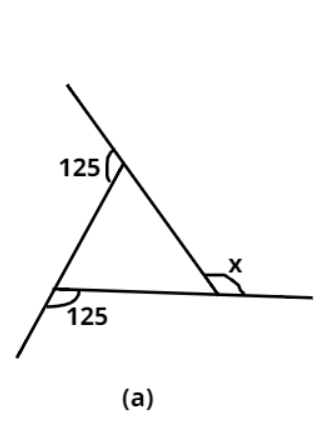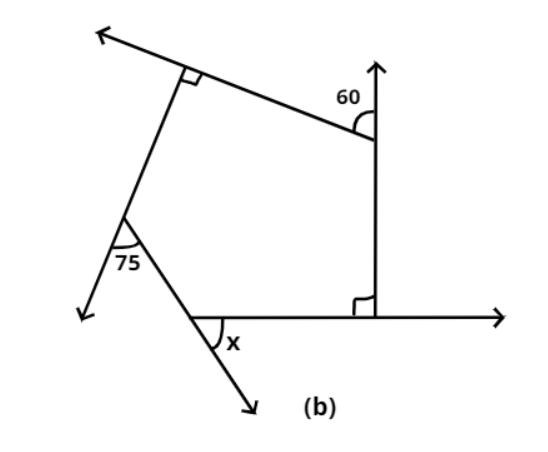Courses
Courses for Kids
Free study material
Free LIVE classes
MoreLIVE
Join Vedantu’s FREE Mastercalss

# Find $x$ in the following figure:Verified
364.8k+ views
Hint: Here we use the exterior angle property. In a polygon Sum of exterior angles is 360.

Complete step by step solution:
We know that , Sum of all exterior angles of any polygon $= {360^ \circ }$

(a) In figure (a) we have,${125^ \circ } + {125^ \circ } + x = {360^ \circ }$
On solving the above equation we get $x$ as
$x = {360^ \circ } - {250^ \circ } = {110^ \circ }$
Hence the value of $x$ is ${110^ \circ }$.

(b) Similarly we do here in figure (b),$x + {75^ \circ } + {60^ \circ } + {90^ \circ } + {90^ \circ } = {360^ \circ }$
On solving we get,
$x = {45^ \circ }$
Hence the value of $x$ is ${45^ \circ }$.

Note: In these types of questions we have to apply properties of exterior angle as the angle we have to find is also exterior. By doing this we can get the correct answer.
Last updated date: 18th Sep 2023
Total views: 364.8k
Views today: 11.64k